Related Articles

# Class 8 NCERT Solutions- Chapter 3 Understanding Quadrilaterals – Exercise 3.3

• Last Updated : 13 Jan, 2021

### Question 1. Given a parallelogram ABCD. Complete each statement along with the definition or property used.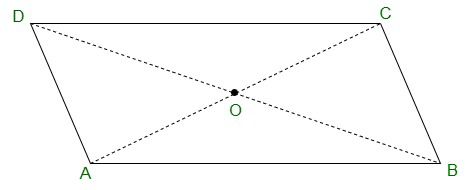Attention reader! All those who say programming isn't for kids, just haven't met the right mentors yet. Join the  Demo Class for First Step to Coding Coursespecifically designed for students of class 8 to 12.

The students will get to learn more about the world of programming in these free classes which will definitely help them in making a wise career choice in the future.

(ii) ∠DCB = ……

(iii) OC = ……

(iv) m ∠DAB + m ∠CDA = ……

Solution:

(i) AD = BC {Opposite sides of a parallelogram are equal}

(ii) ∠DCB = ∠DAB {Opposite angles of a parallelogram are equal}

(iii) OC = OA {Diagonals of a parallelogram are equal}

(iv) m ∠DAB + m ∠CDA = 180°

### Question 2. Consider the following parallelograms. Find the values of the unknown x, y, z

Solution:

(i)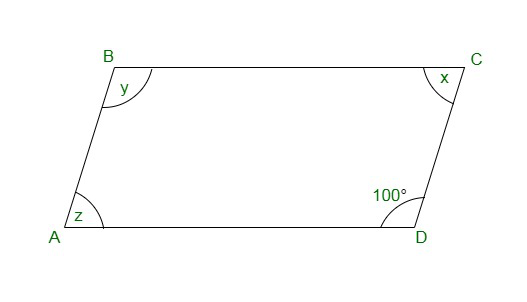y = 100° {opposite angles of a parallelogram}

x + 100° = 180° {Adjacent angles of a parallelogram}

⇒ x = 180° – 100°
⇒ x = 80°

x = z = 80° {opposite angles of a parallelogram}

Therefore,

x = 80°, y = 100° and z = 80°

(ii)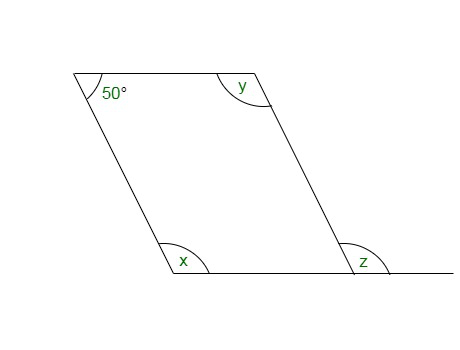50° + x = 180°

⇒ x = 180° – 50° = 130° {Adjacent angles of a parallelogram}

⇒ x = y = 130° {opposite angles of a parallelogram}

⇒ x = z = 130° {corresponding angle}

(iii)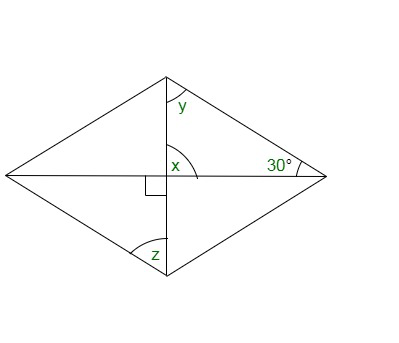x = 90° {vertical opposite angles}

x + y + 30° = 180° {angle sum property of a triangle}

⇒ 90° + y + 30° = 180°

⇒ y = 180° – 120° = 60°

also, y = z = 60° {alternate angles}

(iv)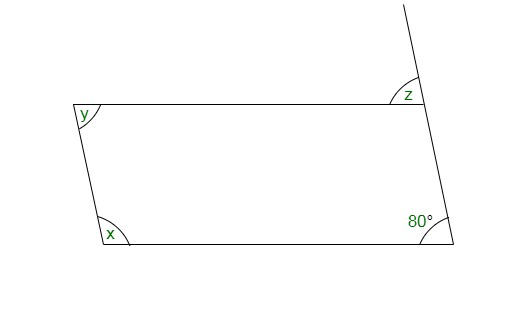z = 80° {corresponding angle}

z = y = 80° {alternate angles}

x + y = 180° {adjacent angles}

⇒ x + 80° = 180°

⇒ x = 180° – 80° = 100°

(v)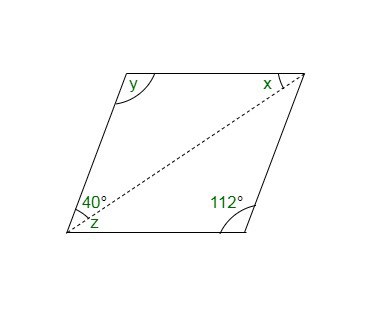y = 112° {opposite angles of a parallelogram}

x = 180° – (y + 40°) {angle sum property of a triangle}

x = 28°

z = 28° {alternate angles}

### Question 3. Can a quadrilateral ABCD be a parallelogram if

(i) ∠D + ∠B = 180°?

(ii) AB = DC = 8 cm, AD = 4 cm and BC = 4.4 cm?

(iii)∠A = 70° and ∠C = 65°?

Solution:

(i) Yes,

The quadrilateral ABCD be a parallelogram if ∠D + ∠B = 180°,

it should also fulfilled some conditions which are:

(a) The sum of the adjacent angles should be 180°.

(b) Opposite angles must be equal.

(ii) No, opposite sides should be of the same length.

(iii) No, opposite angles should be of same measures.

Here, ∠A ≠ ∠C

### Question 4. Draw a rough figure of a quadrilateral that is not a parallelogram but has exactly two opposite angles of equal measure.

Solution: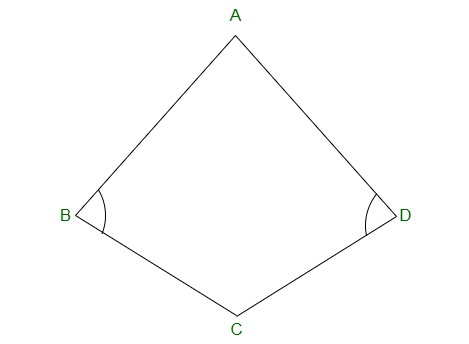ABCD is a figure of quadrilateral which is not a parallelogram but has exactly two opposite angles

that is ∠B = ∠D of equal measure. It is not a parallelogram because ∠A ≠ ∠C.

### Question 5. The measures of two adjacent angles of a parallelogram are in the ratio 3:2. Find the measure of each of the angles of the parallelogram.

Solution:

Let the measures of two adjacent angles ∠A and ∠B be 3x and 2x respectively in parallelogram ABCD.

∠A + ∠B = 180°

⇒ 3x + 2x = 180°

⇒ 5x = 180°

⇒ x = 36°

As we know opposite sides of a parallelogram are equal.

∠A = ∠C = 3x = 3 × 36° = 108°

∠B = ∠D = 2x = 2 × 36° = 72°

### Question 6. Two adjacent angles of a parallelogram have equal measure. Find the measure of each of the angles of the parallelogram.

Solution:

Let ABCD be a parallelogram.

The sum of adjacent angles of a parallelogram = 180°

∠A + ∠B = 180°

⇒ 2∠A = 180°

⇒ ∠A = 90°

also,

90° + ∠B = 180°

⇒ ∠B = 180° – 90° = 90°

∠A = ∠C = 90°

∠B = ∠D = 90°

### Question 7. The adjacent figure HOPE is a parallelogram. Find the angle measures x, y, and z. State the properties you use to find them.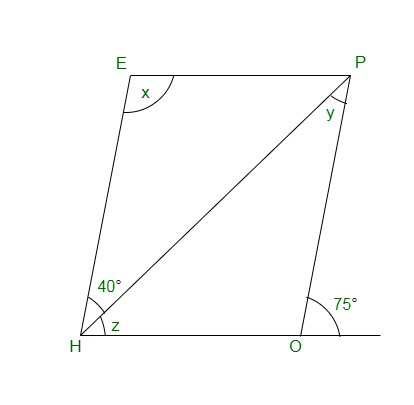Solution:

y = 40° {alternate interior angle}

∠P = 70° {alternate interior angle}

∠P = ∠H = 70° {opposite angles of a parallelogram}

z = ∠H – 40°= 70° – 40° = 30°

∠H + x = 180°

⇒ 70° + x = 180°

⇒ x = 180° – 70° = 110°

### Question 8. The following figures GUNS and RUNS are parallelograms. Find x and y. (Lengths are in cm)

Solution:

(i)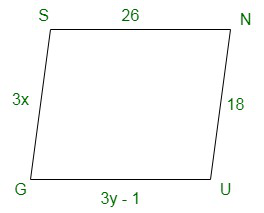SG = NU and SN = GU {opposite sides of a parallelogram are equal}

3x = 18

x = 18/3

⇒ x =6

3y – 1 = 26 and,

⇒ 3y = 26 + 1

⇒ y = 27/3=9

x = 6 and y = 9

(ii)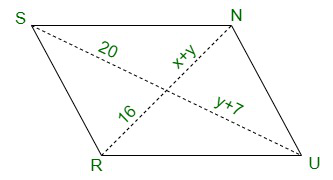20 = y + 7 and 16 = x + y {diagonals of a parallelogram bisect each other}

y + 7 = 20

⇒ y = 20 – 7 = 13 and,

x + y = 16

⇒ x + 13 = 16

⇒ x = 16 – 13 = 3

x = 3 and y = 13

### Question 9. In the above figure, both RISK and CLUE are parallelograms. Find the value of x.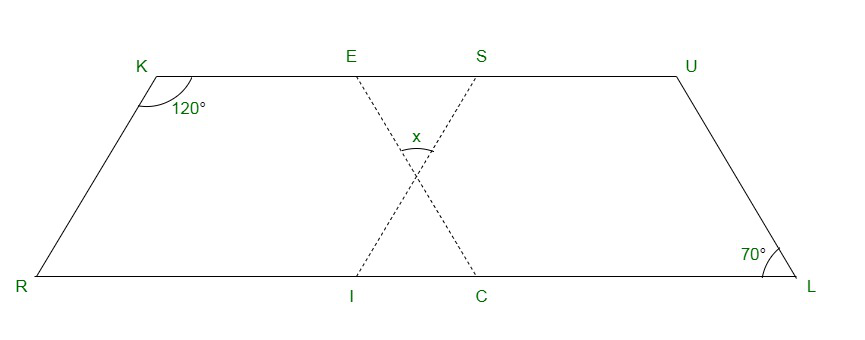Solution:

∠K + ∠R = 180° {adjacent angles of a parallelogram are supplementary}

⇒ 120° + ∠R = 180°

⇒ ∠R = 180° – 120° = 60°

also, ∠R = ∠SIL {corresponding angles}

⇒ ∠SIL = 60°

also,

∠ECR = ∠L = 70° {corresponding angles}

x + 60° + 70° = 180° {angle sum of a triangle}

⇒ x + 130° = 180°

⇒ x = 180° – 130° = 50°

### Question 10. Explain how this figure is a trapezium. Which of its two sides is parallel? (Figure)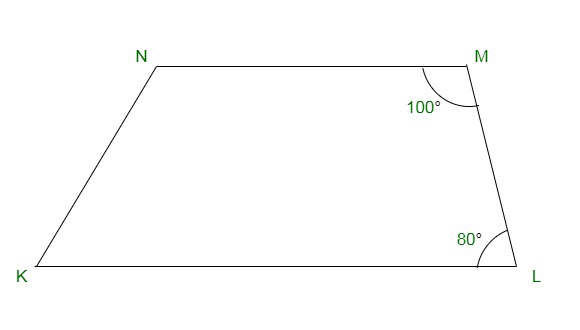Solution:

When a transversal line intersects two lines in such a way that the sum of the adjacent angles on the same side of transversal is 180° then, the lines are parallel to each other.

Here we have, ∠M + ∠L = 100° + 80° = 180°

Hence, MN || LK

As the quadrilateral KLMN has one pair of parallel line therefore it is a trapezium.

MN and LK are parallel lines.

### Question 11. Find m∠C in Figure if AB || DC?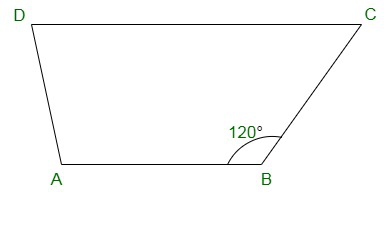Solution:

m∠C + m∠B = 180° {angles on the same side of transversal}

⇒ m∠C + 120° = 180°

⇒ m∠C = 180° − 120° = 60°

### Question 12. Find the measure of ∠P and ∠S if SP || RQ ? in Figure. (If you find m∠R, is there more than one method to find m∠P?)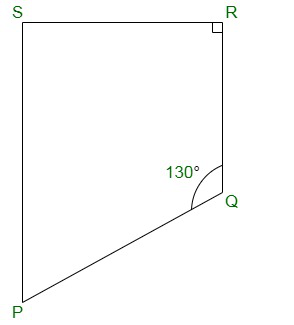Solution:

∠P + ∠Q = 180° {angles on the same side of transversal}

⇒ ∠P + 130° = 180°

⇒ ∠P = 180° – 130° = 50°

also,

∠R + ∠S = 180° {angles on the same side of transversal}

⇒ 90° + ∠S = 180°

⇒ ∠S = 180° – 90° = 90°

Hence, ∠P = 50° and ∠S = 90°

Yes, there are more than one method to find m∠P.

PQRS is a quadrilateral. Sum of measures of all angles of a quadrilateral is 360°.

Thus, as we know the measurement of ∠Q, ∠R and ∠S.

∠Q = 130°, ∠R = 90° and ∠S = 90°

∠P + 130° + 90° + 90° = 360°

⇒ ∠P + 310° = 360°

⇒ ∠P = 360° – 310° = 50°

My Personal Notes arrow_drop_up